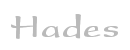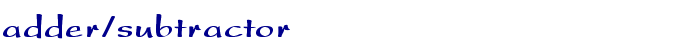TAMS / Java / Hades / applets (print version): contents | previous | nextDescription

A four-bit adder/subtractor demonstration. While it is perfectly possible to design a custom circuit for the subtraction operation, it is much more common to re-use an existing adder and to replace a subtraction by a two-complement's addition.

The applet shows how this is done. Click the input switches or type the 's' bindkey to control the Sub/nAdd switch, and '1' .. '8' to control the A and B inputs.

When the Sub/nAdd input is low (0), the XOR-gates act as non-inverting buffers, and the carry-input to the adder is 0. Therefore, the adder calculates a four-bit sum plus carry-out:

``` (Cout,S3,S2,S1,S0) = (A3,A2,A1,A0) + (B3,B2,B1,B0)
```

If the Sub/nAdd input is high (1), the XOR-gates act as inverting buffers, and the carry-input to the adder is 1.

``` (Cout,S3,S2,S1,S0) = (A3,A2,A1,A0) - (B3,B2,B1,B0)
```

Run the applet | Run the editor (via Webstart)

Impressum | 24.11.06